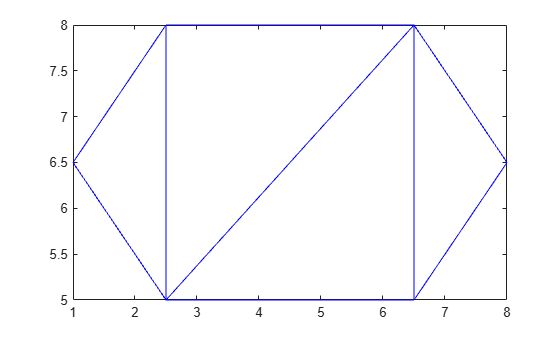# barycentricToCartesian

Convert coordinates from barycentric to Cartesian

## Syntax

``C = barycentricToCartesian(TR,ID,B)``

## Description

example

````C = barycentricToCartesian(TR,ID,B)` returns the Cartesian coordinates of the points in `B` relative to the triangulation object `TR`. Each row of `B` contains the barycentric coordinates of a point with respect to the triangle or tetrahedron indexed by `ID`. The identification numbers of the triangles or tetrahedra in `TR` are the corresponding row numbers of the property `TR.ConnectivityList`.```

## Examples

collapse all

Create a triangulation from a set of points `P` and a triangulation connectivity list `T`, and plot the triangulation.

```P = [2.5 8.0; 6.5 8.0; 2.5 5.0; 6.5 5.0; 1.0 6.5; 8.0 6.5]; T = [5 3 1; 3 2 1; 3 4 2; 4 6 2]; TR = triangulation(T,P); triplot(TR)```Specify the identification number of the first (leftmost) triangle in `TR`, and the barycentric coordinates of the triangle's second point.

```ID = 1; B = [0 1 0];```

Convert the barycentric coordinates to Cartesian coordinates with respect to the first triangle in `TR`.

`C = barycentricToCartesian(TR,ID,B)`
```C = 1×2 2.5000 5.0000 ```

## Input Arguments

collapse all

Triangulation representation, specified as a scalar `triangulation` or `delaunayTriangulation` object.

Data Types: `triangulation` | `delaunayTriangulation`

Triangle or tetrahedron identification, specified as a scalar or a column vector whose elements each correspond to a single triangle or tetrahedron in the triangulation object. The identification number of each triangle or tetrahedron is the corresponding row number of the `ConnectivityList` property.

Data Types: `double`

Barycentric coordinates, specified as a three-column matrix for 2-D coordinates or a four-column matrix for 3-D coordinates.

Data Types: `double`